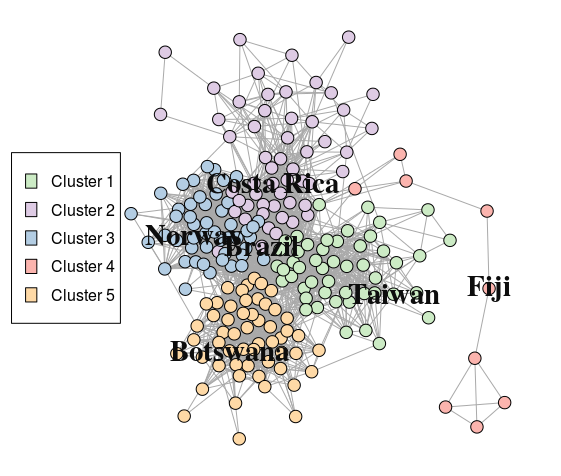# Expected goals from over/under odds

I got a comment the other day asking about whether it is possible to get the expected number of goals scored from over/under odds, similar to how you can do this for odds for win, draw or lose outcomes. The over/under odds refer to the odds for the total score (the sum of the score for two opponents) being over or under a certain value, usually 2.5 in soccer.

It is possible, and rather easy even, to get the expected total score from the over/under odds, at least if you assume that the number of goals scored by the two teams follows a Poisson distribution. This is the same assumption that makes the method for extracting the expected goals from HDW odds possible. The Poisson distribution is really convenient and reasonable realistic probability model for different scorelines. It is controlled by a single parameter, called lambda, that is also the expected value (and the expected goals in this case). One convenient property of the Poisson is that the sum of two Poisson distributed variables with parameters lambda1 and lambda2 is also Poisson distributed, with the lambda being the sum of the two lambdas, i.e. lambdasum = lambda1 + lambda2.

So how can you find the expected total number of goals based on the over/under odds? First you need to convert the odds for the under outcome to a proper probability. How you do this depends on the format your odds come in, but in R you can use the odds.converter package to convert them to decimal format, and then use my own package called implied to convert them to proper probabilities.

After you have the probabilities for the under probability, you can use the Poisson formula to find the value of the parameter lambda that gives the probability for the under outcome that matched the probability from the odds. In R you can use the built-in ppois function to compute the probabilities for there being scored less than 2.5 goals when the expected total goals is 3.1 like this:

under <- 2.5
ppois(floor(under), lambda=3.1)


This will give us that the probability is 40.1% of two or less goals being scored in total, when the expected total is 3.1. Now you can try to manually adjust the lambda parameter until the output matches your probability from the odds. Another way is to automate this search using the built-in uniroot function. The uniroot function takes as input another function, and searches for the input value that gives the result 0. We therefore have to write a function that takes as input the expected goals, the probability implied by the odds, and the over/under limit, and returns the difference between the probability from the Poisson model and the odds probability. Here is one such function:

obj <- function(expg, under_prob, under){
(ppois(floor(under), lambda=expg) - under_prob)
}


Next we feed this to the uniroot function, and gives a realistic search interval for the expected goals, between 0.01 and 10 in this case, and the rest of the parameters. For this example I used 62% chance of there being scored less than 2.5 goals.

uniroot(f = obj,
interval = c(0.01, 10),
under_prob = 0.62,
under = 2.5)


From this I get that the expected total goals is 2.21.

You might wonder if it is possible to get the separate expected goals for the two teams from over/under odds using this method. This is unfortunately not possible. The only thing you can hope for is to get a range of possible values for the two expected goals that sums to the total expected goals. In our example with the expected total goals being 2.21, the range of possible values for the two expected goals can be plotted as a line like this:If course, you can judge some pairs of expected goals being more likely than others, but there is no information about this in over/under odds alone. It might be possible, I am not 100% sure, that other non-Poisson models, which would involve more assumptions, could exploit the over/under odds to get expected goals for both teams.

# The probabilities implied by bookmaker odds: Introducing the ‘implied’ package

My package for converting bookmaker odds into probabilities is now on available from CRAN. The package contains several different conversion algorithms, which are all accessible via the implied_probabilities() function. I have written an introduction on how you can use the package here, together with a description of all the methods and with references to papers. But I also want to give some background to some of the methods here on the blog as well.

In statistics, an odd is usually taken to mean the inverse of a probability, that is 1/p, but in the betting world different odds formats exists. As usual, Wikipedia has a nice overview of the different formats. In the implied package, only inverse probability odds are allowed as inputs, which in betting are called decimal odds.

Now you might think that converting decimal odds to probabilities should be easy, you can just use the definition above and take the inverse of the odds to recover the probability. But it is not that simple, since in practice using this simple formula will give you improper probabilities. They will not sum to 1, as they should, but be slightly larger. This gives the bookmakers an edge and the probabilities (which aren’t real probabilities) can not be considered fair, and so different methods for correcting this exists.

Some methods uses different types of regression modelling combined with historical data to estimate the biases in the different outcomes. This is for example the case in the paper On determining probability forecasts from betting odds by Erik Štrumbelj. Anyway, the implied package does not include these kinds of methods. The reason I wanted to mention this paper is that this was where I first read about Shin’s method for the first time.

All the methods in the package are what I call one-shot methods. The conversion of a set of odds for a game only relies on the odds them self, and not on any other data. This is deliberate choice, since I didn’t want to make a modelling package, since that would be much more complicated.

Many of the methods in the package comes are described in the Wisdom of the Crowd document by Joseph Buchdahl, and a review paper by Clarke et al (Adjusting Bookmaker’s Odds to Allow for Overround).

Many of the methods in the package can be described as ad hoc methods. They basically use a simple mathematical formula that relates the true underlying probabilities to the improper probabilities given by the bookmakers odds. Then this formula is used to find the true probabilities so that they are proper (sum to 1) while also recovering the improper bookmaker probabilities.

A few other methods in the package are more theory based, like Shin’s method, and I find these methods really interesting. Shin’s method imagine that there are two types of bettors. The first type is the typical bettor, and the sum of bets by this type follows the “wisdom of the crowd” pattern which should reflect the true ncertainty of the outcome given the publicly available information. Then there is a second type of bettor, which has inside information and always bets on the winning outcome. However, the bookmaker don’t know what type of bettor the individual bettors are, and only observes the mixture of the two types. Here is the interesting part: By assuming the bookmakers know that there are two types of bettors, and that the bookmakers seek to maximize their profits, Shin was able to derive some complicated formulas that relate the true underlying “wisdom of the crowds” probabilities and the bookmakers odds. These formulas can be used in the same way as the ad hoc methods to find the underlying probabilities.

A natural question question is what method gives the most realistic probabilities? There is no definite answer to this, and different methods will be best in different markets and settings. You need to figure this out for yourself.

I am currently working on some new methods inspired by Shin’s framework which I hope to write about later. Shin’s work was mostly done in the context of horse racing, where there is realistic that some bettors have inside information. I hope to develop a method that is more relevant for football.

# Expected goals from bookmaker odds

I recently read an interesting paper called The Betting Odds Rating System: Using soccer forecasts to forecast soccer by Wunderlich and Memmert. In their paper they develop av variant of the good old Elo rating system. Instead of using the actual outcomes of each match to calculate the ratings, they use the probabilities of the outcomes, which they get from bookmaker odds.

I was wondering if a similar approach could be used together with the goalmodel package I released a couple of months ago. The models available in the package are models I have written about extensively on this blog, and they all work as follows: You use the number of goals scored to get some ratings of the goal scoring and goal conceding rates of each team. You then use these ratings to forecast the expected number of goals in the upcoming games. These expected goals can then be used to calculate the probabilities of the outcome (Home win, draw, away win). A crucial step in these calculations is the assumption that the number of goals scored follow the Poisson distribution (or some related distribution, like the Negative Binomial).

But can we turn this process the other way around, and use bookmaker odds (or odds from other sources) to get expected goals and maybe also attack and defense ratings like we do in the goalmodel package? I think this is possible. I have written a function in R that takes outcome probabilities and searches for a pair of expected goals that matches the probabilities. You can find it on github (Edit: The function is now included in the goalmodel package.). This function relies on using the Poisson distribution.

Next, I have expanded the functionality of the goalmodel package so that you can use expected goals for model fitting instead of just observed goals. This is possible by setting the model argument to “model = ‘gaussian'” or to “model = ‘ls'”. These two options are currently experimental, and are a bit unstable, so if you use them, make sure to check if the resulting parameter estimates make sense.

I used my implied package to convert bookmaker odds from the 2015-16 English Premier League into probabilities (using the power method), found the expected goals, and then fitted a goalmodel using the least squares method. Here are the resulting parameters, from both using the expected goals and observed goals: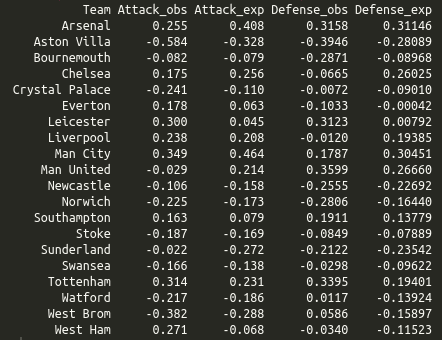I wanted to use this season for comaprison as this was the season Leicester won unexpectedly, and in the Odds-Elo paper (figure 6) it seemed like the ratings based on the odds were more stable than the ones based on the actual results, which increased drastically during the season. In the attack and defense ratings from the goalmodels we see that Leicester have average ratings (which is what ratings close to 0 are) in the model based on odds, and much higher ratings based on the actual results. So the goalmodel and Elo ratings seem to agree, basically.

I also recently discovered another paper titled Combining historical data and bookmakers’odds in modelling football scores, that tries something similar as I have done here. They seem to do the same extraction of the expected goals from the bookmaker odds as I do, but they don’t provide the details. Instead of using the expected goals to fit a model, they fit a model based on actual scores (similar to what the goalmodel package do), and then they take a weighted average of the model based expected goals and the expected goals from the bookmaker odds.

# Introducing the goalmodel R package

I have written a lot about different models for forecasting football results, and provided a lot of R code along the way. Especially popular are my posts about the Dixon-Coles model, where people still post comments, four years since I first wrote them. Because of the interest in them, and the interest in some of the other models I have written about, I decided to tidy up my code and functions a bit, and make an R package out of it. The result is the goalmodel R package. The package let you fit the ordinary Poisson model, which was one of the first models I wrote about, the Dixon-Coles model, The Negative-Binomial model, and you can also use the adjustment I wrote about in my previous update.

The package contains a function to fit the different models, and you can even combine different aspects of the different models into the same model. You can for instance use the Dixon-Coles adjustment together with a negative binomial model. There is also a range of different methods for making prediction of different kinds, such as expected goals and over/under.

The package can be downloaded from github. It is still just the initial version, so there are probably some bugs and stuff to be sorted out, but go and try it out and let me know what you think!

# A small adjustment to the Poisson model that improves predictions.

There are a lot extensions to the basic Poisson model for predicting football results, where perhaps the most popular is the Dixon-Coles model which I and other have written a lot about. One paper that seem to have received little attention is the 2001 paper Prediction and Retrospective Analysis of Soccer Matches in a League by Håvard Rue and Øyvind Salvesen (preprint available here). The model they describe in the paper extend the Dixon-Coles and Poisson model in several ways. The most interesting extension in how they allow the attack and defense parameters vary over time, by estimating a separate set of parameters for each match. This might at first seem like a task that should be impossible, but they manage to pull it of by using some Bayesian magic that let the estimated parameters borrow information across time. I have tried to implement something similar like this in Stan, but I haven’t gotten it to work quite right, so that will have to wait for another time. There’s many other interesting extensions in the paper as well, and here I am going to focus on one of of them which is an adjustment for teams to over and underestimate opponents when they differ in strengths.

The adjustment is added to the formulas for calculating the log-expected goals. So if team A plays team B at home, the log-expected goals $$\lambda_A$$ and $$\lambda_B$$

$$\lambda_A = \alpha + \beta + attack_{A} – defense_{B} – \gamma \Delta_{AB}$$

$$\lambda_B = \alpha + attack_{B} – defense_{A} + \gamma \Delta_{AB}$$

In these formulas are $$\alpha$$ the intercept, $$\beta$$ the home team advantage and $$\Delta_{AB}$$ is a factor that determines the amount a team under- or overestimation the strength of the opponent. This factor is given as

$$\Delta_{AB} = (attack_{A} + defense_{A} – attack_{B} – defense_{B}) / 2$$

The parameter $$\gamma$$ determines how large this effect is. A positive $$\gamma$$ implies that a strong team will underestimate a weak opponent, and thereby score fewer goals than we would otherwise expect, and vice versa for the opponent.

In the paper they do not estimate the $$\gamma$$ parameter directly together with the other parameters, but instead set it to a constant, with a value they determine by backtesting to maximize predictive ability.

When I implemented this model in R and estimated it using Maximum Likelihood I noticed that adding the adjustment did not improve the model fit. I suspect that this might be because the model is nearly unidentifiable. I even tried to add a Normal prior on $$\gamma$$ and get a Maximum a Posteriori (MAP) estimate, but then the MAP estimate were completely determined by the expected value of the prior. Because of these problems I decided to use a different strategy: I estimated the model without the adjustment, but add the adjustment when making predictions.

I am not going to post any R code on how to do this, but if you have estimated a Poisson or Dixon-Coles model, it should not be that difficult to add the adjustment when you calculate the predictions. If you are going to use some of the code I have posted on this blog before, you should notice the important detail that in the formulation above I have followed the paper and changed the signs of the defense parameters.

In the paper Rue and Salvesen write that $$\gamma = 0.1$$ seemed to be an overall good value when they analyze English Premier League data. To see if my approach of adding the adjustment only when doing predictions is reasonable I did a leave-one-out cross validation on some seasons of English Premier League and German Bundesliga. I fitted the model to all the games in a season, except one, and then add the adjustment when predicting the result of the left out match. I did this for several values of $$\gamma$$ to see which values works best.

Here is a plot of the Ranked Probability Score (RPS), which is a measure of prediction accuracy, against different values of $$\gamma$$ for the 2011-12 Premier League season:As you see I even tried some negative values of $$\gamma$$, just in case. At least in this season the result agrees with the estimate $$\gamma = 0.1$$ that Rue and Salvesen reported. In some of the later seasons that I checked the optimal $$\gamma$$ varies somewhat. In some seasons it is almost 0, but then again in some others it is around 0.1. So at least for Premier league, using $$\gamma = 0.1$$ seems reasonable.

Things are a bit different in Bundesliga. Here is the same kind of plot for the 2011-12 season: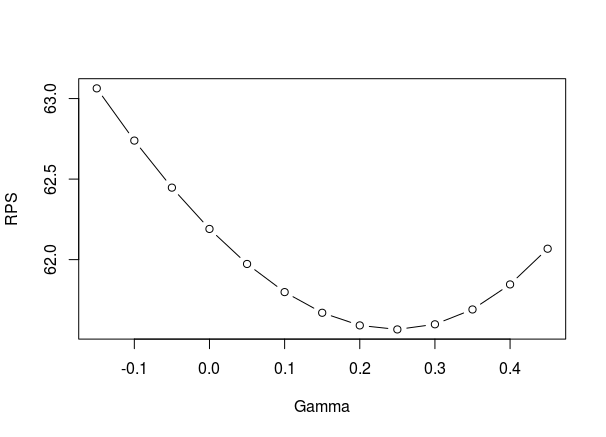As you see the optimal value here is around 0.25. In the other seasons I checked the optimal value were somewhere between 0.15 and 0.3. So the effect of over- and underestimating the opponent seem to be greater in the Bundesliga than in Premier League.

# A simple re-implementation of the Dixon-Coles model

A couple of years ago I implemented the Dixon-Coles model for predicting football results here on this blog. That series of of blog posts is my most popular since I keep getting comments on it, some four years later.

One of the most common requests is advice on how to expand the model to include additional predictors. Unfortunately with the implementation I posted this was not so straightforward. It relied on some design matrices with dummy-coded variables, which is a standard way of doing things in regression modeling. The DC model isn’t a standard regression modeling problem, so using matrices complicated things. I posted some updates and variant across several posts, which in the end made the code a bit hard to follow and to modify.

Anyway, I’ve had a simpler implementation lying around for a while, and since there’s been far between updates on this blog lately I thought I’d post it.

First load some data from the engsoccerdata package. I’m going to use the 2011-12 season of the English Premier League, so the results can be compared with what I got from the first implementation.

library(dplyr)
library(engsoccerdata)

england %>%
filter(Season == 2011,
tier==1) %>%
mutate(home = as.character(home),
visitor = as.character(visitor))-> england_2011


Next we should create a list of initial parameter values. This will be used as a starting point for estimating the parameters. The list contains vectors of four groups of parameters, the attack and defense parameters of all teams, the home field advantage and the Dixon-Coles adjustment (rho). The attack and defense vector are named so that it is easy to look up the relevant parameter later on.

Notice also that a sum-to-zero constraint has to be added to the defense parameters, so in reality we are estimating one defense parameter less than the number of teams. Check this post for some more explanation of this.

# Make a vector of all team names.
all_teams <- sort(unique(c(england_2011$home, england_2011$visitor)), decreasing = FALSE)
n_teams <- length(all_teams)

# list of parameters with initial values.
parameter_list <- list(attack = rep(0.2, n_teams),
defense = rep(-0.01, n_teams-1),
home = 0.1,
rho= 0.00)

names(parameter_list$attack) <- all_teams names(parameter_list$defense) <- all_teams[-1] # the first parameter is computed from the rest.


Next we need a function that calculates the negative log-likelihood function, to be used with R’s built in optimizer.

One trick I use here is to relist the parameters. The optimizer want all parameter values as a single vector. When you have a lot of parameters that group together and is used in different parts of the model, this can quickly create some complicated indexing and stuff. By supplying the original parameter list, plus having named vectors, these problems essentially disappear.

Also notice how the expected goals are now simply computed by looking up the relevant parameters in the parameter list and adding them together. No need for matrix multiplications.

The Dixon-Coles adjustment function tau is the same as in the original implementation.

dc_negloglik <- function(params, goals_home, goals_visitor,
team_home, team_visitor, param_skeleton){

# relist, to make things easier.
plist <- relist(params, param_skeleton)

# There is a sum-to-zero constraint on defense parameters.
# The defense parameter for the first team is computed from the rest.
plist$defense <- c(sum(plist$defense)*-1, plist$defense) names(plist$defense) <- names(plist$attack) # add name to first element. # Home team expected goals lambda_home <- exp(plist$attack[team_home] + plist$defense[team_visitor] + plist$home)

# Away team expected goals
lambda_visitor <- exp(plist$attack[team_visitor] + plist$defense[team_home])

# Dixon-Coles adjustment
dc_adj <- tau(goals_home, goals_visitor, lambda_home, lambda_visitor, rho = plist$rho) # Trick to avoid warnings. if (any(dc_adj <= 0)){ return(Inf) } # The log-likelihood log_lik_home <- dpois(goals_home, lambda = lambda_home, log=TRUE) log_lik_visitor <- dpois(goals_visitor, lambda = lambda_visitor, log=TRUE) log_lik <- sum((log_lik_home + log_lik_visitor + log(dc_adj))) return(log_lik*-1) }  To actually estimate the parameters we feed the function, data and initial values to optim, and check the results.  optim_res <- optim(par = unlist(parameter_list), fn=dc_negloglik, goals_home = england_2011$hgoal,
goals_visitor = england_2011$vgoal, team_home = england_2011$home, team_visitor = england_2011$visitor, param_skeleton=parameter_list, method = 'BFGS') # relist, and calculate the remaining parameter. parameter_est <- relist(optim_res$par, parameter_list)
parameter_est$defense <- c( sum(parameter_est$defense) * -1, parameter_est$defense) names(parameter_est$defense) <- names(parameter_est\$attack)


I get the same home field advantage (0.27) and rho (-0.13) as in the original implementation. The other parameters differ, however. This is because of the sum-to-zero constraints are coded in a different way. This should not matter and both ways should give the same predictions.

I have not yet said anything about how to expand the model to include other predictors, but hopefully this implementation should make it easier. You can just add some new arguments to the dc_negloglik function that takes the variables in question as input, and add new parameter vectors to the parameter list as needed. Then the calculations of the expected goals should be modified to include the new parameters and predictors.

# The Bayesian Bradley-Terry model with draws

In the previous post I tried out the Stan software to implement two Bayesian versions of the Bradley-Terry (BT) model. One drawback of the Bradley-Terry model is that it can’t handle draws, which seriously hampers its utility in modelling sports data. That was one reason I used handball results rather than football results as the example, since draws are rare in handball.

One (of several) extension of the BT model that can handle draws is the Davidson model. This was developed in the 1970 paper ‘On Extending the Bradley-Terry Model to Accommodate Ties in Paired Comparison Experiments‘. In short, the model adds a new parameter, $$\nu$$, which influences the probability of a draw. When $$\nu = 0$$, the model becomes the ordinary BT model.

In my Stan implementation below I use a Dirichlet prior on the ratings, like last time. The consequence of this is that the sum of all the ratings is 1. In the BT model this gives us the the nice interpretation that the rating the probability of a team of winning against an hypothetical average team. This property is not exactly carried over to the Davidson model, but a related property is. The ratio of two ratings, $$\pi_1 / \pi_2$$, is the probability that team 1 wins against team 2, applies to both the BT model and the Davidson model

In my implementation of the BT model I used the Bernoulli distribution to model the outcomes, which is appropriate when we only have two outcomes. As you can see from the code below, we now have to use the categorical distribution, since we now have three outcomes. I also use an exponential prior on $$\nu$$. Admittedly, I have no particular reason for this except that it is the traditional choice for parameters that has to have only positive values.

Anyway, here is the Stan code:

data {
int<lower=0> N; // N games
int<lower=0> P; // P teams

// Each team is referred to by an integer that acts as an index for the ratings vector.
int team1[N]; // Indicator arrays for team 1
int team2[N]; // Indicator arrays for team 1
int results[N]; // Results. 1 if home win, 2 if away won, 3 if a draw.

real<lower=0> nu_prior_rate;

vector[P] alpha; // Parameters for Dirichlet prior.
}

parameters {
// Vector of ratings for each player
// The simplex constrains the ratings to sum to 1
simplex[P] ratings;

// Parameter adjusting the probability of draw.
real<lower=0> nu;
}

model {

// Array of length 3 vectors for the three outcome probabilies for each game.
vector result_probabilities[N];
real nu_rating_prod;

ratings ~ dirichlet(alpha); // Dirichlet prior on the ratings.

nu ~ exponential(nu_prior_rate); // exponential prior on nu.

for (i in 1:N){

// nu multiplied by the harmonic mean of the ratings.
nu_rating_prod = sqrt(ratings[team1[i]] * ratings[team2[i]]) * nu;

result_probabilities[i] = nu_rating_prod / (ratings[team1[i]] + ratings[team2[i]] + nu_rating_prod);
result_probabilities[i] = ratings[team1[i]] / (ratings[team1[i]] + ratings[team2[i]] + nu_rating_prod);
result_probabilities[i] = 1 - (result_probabilities[i] + result_probabilities[i]);

results[i] ~  categorical(result_probabilities[i]);
}

}


Another thing I wanted to do this time was to do proper MCMC sampling, so we could get the Bayesian posterior credibility intervals. The sampling takes longer time than the optimization procedure I used last time, but it only took a few seconds to get a decent amount of samples.

For the reanalysis of the handball data from last time I set the Dirichlet prior parameters to 5 for all teams, and the rate parameter for the exponential prior on $$\nu$$ is 1. We can visualize the estimate of the ratings and their uncertainties (95% intervall) using a forest plot:The results agree with the ones from last time, but this time we also see that the credibility intervals are rather large. This is perhaps not that surprising, since the amount of data is rather limited. The posterior (mean) point estimate for $$\nu$$ is 0.15.

But let’s take a look at some English Premier League football data. With the ordinary BT model this would not work so well since there’s a lot of draws in football. Ignoring them would not be tenable. Below are the ratings, with 95% credibility interval, based on data from the 2015-15 season, using the same prior parameters as in the handball data set. The league points are shown in parenthesis for comparison.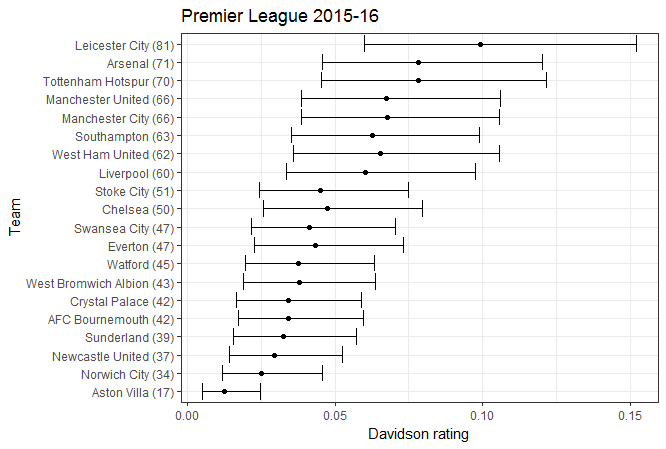The ratings generally agree with the points, except in a few instances, where a team or two have switched places. Another interesting thing to notice is that the width of the intervals seem to be related to the magnitude of the rating. I am not exactly sure why that is, but I suspect its due to the fact that the ratings are in a sense binomial probabilities, and these are known to have greater variance the closer they are to 0.5.

The point estimate for $$\nu$$ is 0.85 for this data set. Compared to the 0.15 for the handball data, it is clear that this reflects the higher overall probability of draws in football. In the handball data set only 6 games ended in a draw, while in the football data set about 20% of the games was a draw.

# Fitting Bradley-Terry models using Stan

I have recently played around with Stan, which is an excellent software to fit Bayesian models. It is similar to JAGS, which I have used before to fit some regression models for predicting football results. Stan differs from JAGS in a number of ways. Although there is some resemblance between the two, the model specification languages are not compatible with each other. Stan, for instance, uses static typing. On the algorithmic side, JAGS uses the Gibbs sampling technique to sample from the posterior; Stan does not do Gibbs sampling, but has two other sampling algorithms. In Stan you can also get point estimates by using built-in optimization routines that search for the maximum of the posterior distribution.

In this post I will implement the popular Bradley-Terry machine learning model in Stan and test it on some sports data (handball, to be specific).

The Bradley-Terry model is used for making predictions based on paired comparisons. A paired comparison in this context means that two things are compared, and one of them is deemed preferable or better than the other. This can for example occur when studying consumer preferences or ranking sport teams.

The Bradley-Terry model is really simple. Suppose two teams are playing against each other, then the probability that team i beats team j is

$$p(i > j) = \frac{r_i}{r_i + r_j}$$

where $$r_i$$ and $$r_j$$ are the ratings for the two teams, and should be positive numbers. It is these ratings we want to estimate.

A problem with the model above is that the ratings are not uniquely determined. To overcome this problem the parameters need to be constrained. The most common constraint is to add a sum-to-one constraint

$$\sum_k r_k = 1$$

I will explore a different constraint below.

Sine we are in a Bayesian setting we need to set a prior distribution for the rating parameters. Given the constraints that the parameters should be positive and sum-to-one the Dirichlet distribution is a natural choice of prior distribution.

$$r_1, r_2, …, r_p \sim Dir(\alpha_1, \alpha_2, …, \alpha_p)$$

where the hyperparameters $$\alpha$$ are positive real numbers. I will explore different choices of these below.

Here is the Stan code for the Bradley-Terry model:

data {
int<lower=0> N; // N games
int<lower=0> P; // P teams

// Each team is referred to by an integer that acts as an index for the ratings vector.
int team1[N]; // Indicator arrays for team 1
int team2[N]; // Indicator arrays for team 1
int results[N]; // Results. 1 if team 1 won, 0 if team 2 won.

vector[P] alpha; // Parameters for Dirichlet prior.
}

parameters {
// Vector of ratings for each team.
// The simplex constrains the ratings to sum to 1
simplex[P] ratings;
}

model {
real p1_win[N]; // Win probabilities for player 1

ratings ~ dirichlet(alpha); // Dirichlet prior.

for (i in 1:N){
p1_win[i] = ratings[team1[i]] / (ratings[team1[i]] + ratings[team2[i]]);
results[i] ~ bernoulli(p1_win[i]);
}
}


The way I implemented the model you need to supply the hyperparameters for the Dirichlet prior via R (or whatever interface you use to run Stan). The match outcomes should be coded as 1 if team 1 won, 0 if team 2 won. The two variables team1 and team2 are vectors of integers that are used to reference the corresponding parameters in the ratings parameter vector.

Before we fit the model to some data we need to consider what values we should give to the hyperparameters. Each of the parameters of the Dirichlet distribution corresponds to the rating of a specific team. Both the absolute magnitude and the relative magnitudes are important to consider. A simple case is when all hyperparameters have the same value. Setting all hyperparameters to be equal to each other, with a value greater or equal to 1, implies a prior belief that all the ratings are the same. If they are between 0 and 1, the prior belief is that the ratings are really really different. The magnitude also plays a role here. The greater the magnitudes are, the stronger the prior belief that the ratings are the same.

Let’s fit some data to the model. Below are the results from fitting the results from 104 games from the 2016-17 season of the Norwegian women’s handball league, with 11 participating teams. I had to exclude six games that ended in a tie, since that kind of result is not supported by the Bradley-Terry model. Extension exists that handle this, but that will be for another time.

Below are the results of fitting the model with different sets of priors, together with the league points for comparison. For this purpose I didn’t do any MCMC sampling, I only found the MAP estimates using the optimization procedures in Stan.For all the priors the resulting ratings give the same ranking. This ranking also corresponds well with the ranking given by the league points, except for Gjerpen and Stabæk which have switched place. We also clearly see the effect of the magnitude of the hyperparameters. When all the $$\alpha$$‘s are 1 the ratings varies from almost 0 to about 0.6. When they are all set to 100 the ratings are almost all the same. If these ratings were used to predict the result of future matches the magnitudes of the hyperparameters could be tuned using cross validation to find the value that give best predictions.

What if we used a different hyperparameter for each team? Below are the results when I set all $$\alpha$$‘s to 10, except for the one corresponding to the rating for Glassverket, which I set to 25. We clearly see the impact. Glassverket is now considered to be the best team. This is nice since it demonstrates how actual prior information, if available, can be used to estimate the ratings.I also want to mention another way to fit the Bradley-Terry model, but without the sum-to-one constraint. The way to do this is by using a technique that the Stan manual calls soft centering. Instead of having a Dirichlet prior which enforces the constraint, we use a normal distribution prior. This prior will not give strict bounds on the parameter values, but will essentially provide a range of probable values they can take. For the model I chose a prior with mean 20 and standard deviation 6.

$$r_1, r_2, …, r_p \sim N(\mu = 20, \sigma = 6)$$

The mean hyperprior here is arbitrary, but the standard deviation required some more considerations. I reasoned that the best team would probably be in the top 99 percentile of the distribution, approximately three standard deviations above the mean. In this case this would imply a rating of 20 + 3*6 = 38. Similarly, the worst team would probably be rated three standard deviations below the mean, giving a rating of 2. This implies that the best team has a 95% chance of winning against the worst team.

Here is the Stan code:

data {
int<lower=0> N;
int<lower=0> P;

int team1[N];
int team2[N];
int results[N];

real<lower=0> prior_mean; // sets the (arbitrary) location of the ratings.
real<lower=0> prior_sd; // sets the (arbitrary) scale of the ratings.

}

parameters {
real<lower=0> ratings[P];
}

model {
real p1_win[N];

// soft centering (see stan manual 8.7)
ratings ~ normal(prior_mean, prior_sd);

for (i in 1:N){
p1_win[i] = ratings[team1[i]] / (ratings[team1[i]] + ratings[team2[i]]);
results[i] ~ bernoulli(p1_win[i]);
}
}


And here are the ratings for the handball teams. The ratings are now on a different scale than before and largely matches the prior distribution. The ranking given by this model agrees with the model with the Dirichlet prior, with Gjerpen and Stabek switched relative to the league ranking.# Which model is the best?

I had a discussion on Twitter a couple of weeks ago about which model is the best for predicting football results. I have suspected that the Dixon & Coles model (DC), which is a modification of the Poisson model, tend to overfit. Hence it should not generalize well and give poorer predictions. I have written about one other alternative to the Poisson model, namely the Conway-Maxwell Poisson model (COMP). This is a model for count data that can be both over-, equi- and underdispersed. It is basically a Poisson model but without the assumption that the variance equals the mean. I have previously done some simple analyses comparing the Poisson, DC and COMP models, and concluded then that the COMP model was superior. The analysis was however a bit to simple, so I have now done a more proper evaluation of the models.

A proper way to evaluatie the models is to do a backtest. For each day there is a game played, the three models are fitted to the available historical data (but not data from the future, that would be cheating) and then used to predict the match outcomes for that day. I did this for two leagues, the English Premier League and German Bundesliga. The models were fitted to data from both the top league and the second tier divisions, since this improves the models, but only the results of the top division was predicted and used in the evaluation. I used a separate home field advantage for the two divisions and the rho parameter in the DC model and the dispersion parameter in the COMP model was estimated using the top division only.

To measure the model’s predictive ability I used the Ranked Probability Score (RPS). This is the proper measure to evaluate predictions for the match outcome in the form of probabilities for home win, draw and away win. The range of the RPS goes from 0 (best possible predictions) to 1 (worst possible prediction). Since the three models actually model the number of goals, I also looked at the probability they gave for the actual score.

For all three models I used the Dixon & Coles method to weight the historical data that is used in training the models. This requires tuning. For both the English and German leagues I backtested the models on different values of the weighting parameter $$\xi$$ on the seasons from 2005-06 to 2009-10, with historical data available from 1995. I then used the optimal $$\xi$$ for backtesting the seasons 2010-11 up to December 2016. This last validation period covers 1980 Bundesliga matches and 2426 Premier League matches.

Here are the RPS for the three models plottet against $$\xi$$. Lower RPS is better and lower $$\xi$$ weights more recent data higher.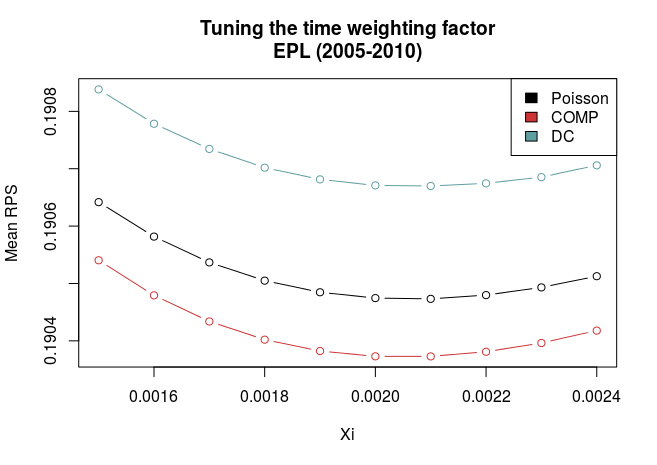The graphs show a couple of things. First, all three models have best predictive ability at the same value of $$\xi$$, and that they compare similarly also for non-optimal values of $$\xi$$. This makes things a bit easier since we don’t have to worry that a different value of $$\xi$$ will alter our evaluations about which model is the best.

Second, there is quite some difference between the models for the German and English data. In the English data the COMP model is clearly best, while the DC is the worst. In the German league, the DC is clearly better, and the COMP and Poisson models are pretty much equally good.

So I used the optimal values of $$\xi$$ (0.0021 and 0.0015 for Premier League and Bundesliga, respectively) to validate the models in the data from 2010 and onwards.

Here is a table of the mean RPS for the three models: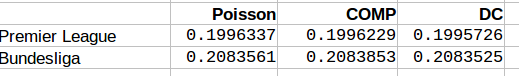We see that for the both English Premier League and German Bundesliga the DC model offers best predictions. The COMP model comes second in Premier League, but has worst performance in the Bundesliga. It is interesting that the DC model performed worst in the tuning period for the Premier League, now was the best one. For the Bundesliga the models compared similarly as in the tuning period.

I also looked at how often the DC and COMP models had lower RPS than the Poisson model. The results are in this table: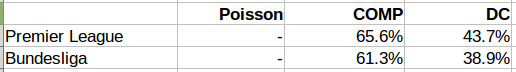The COMP model outperformed the Poisson model in more than 60% of the matches in both leagues, while the DC model did so only about 40% of the time.

When looking at the goal scoring probabilities. Here is a table of the sum of the minus log probabilities for the actual scoreline. Here a lower number also indicates better predictions.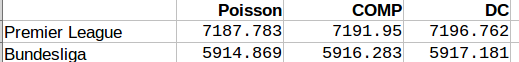Inn both the Premier League and Bundesliga the Poisson model was best, followed by COMP, with the DC model last.

We can also take a look at the parameter values for the extra parameters the DC and COMP models has. Remember that the DC models is becomes the Poisson model when rho = 0, while the COMP model is the same as the Poisson model when upsilon = 1, and is underdispersed when upsilon is greater than 1.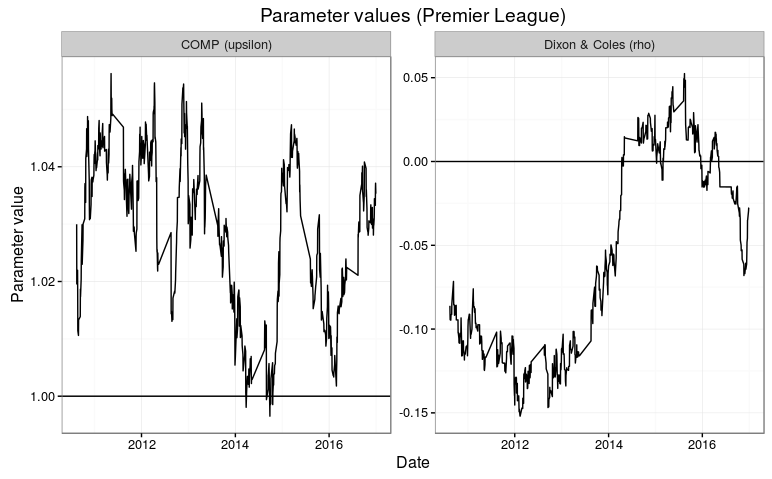The parameter estimates fluctuates a bit. It is intersting to see that the rho parameter in the DC model tend to be below 1, which gives the opposite direction of what Dixon and Coles found in their 1997 paper. In the Premier League, the parmater makes a big jump to above 0 at the end of the 2013-14 season. The parameter appears to be a bit more consistent in the Bundesliga, but also there we see a short period where the parameter is around 0.

The dispseriosn parameter upsilon also isn’t all that consistent. It is generally closer to 1 in the Bundesliga than in the Premier League. I think this is consistent with why this model was better in the Premier League than in the Bundesliga.

All inn all I think it is hard to conclude which of the three models is the best. The COMP and DC models both adjusts the Poisson model in their own specific ways, and this may explain why the different ways of measuring their predictive abilities are so inconsistent. The DC model seem to be better in the German Bundesliga than in the English Premier League. I don’t think any of the two models are generally better than the ordinary Poisson model, but it could be worthwhile to look more into when the two models are better, and perhaps they could be combined?

# The comparison graph part 2

In the last post I wrote about how a graph could be used to explore an important aspect of a data set of football matches, namely whom has played against whom. In this post I will present a more interesting graph. Here is how a graph of 4500 international matches, including friendlies, world cups, and continental cups, from 2010 to 2015: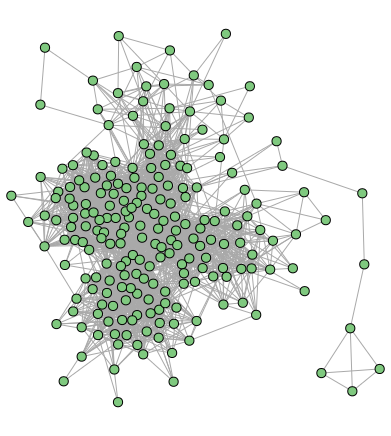There are 214 teams in this data set, each represented by a circle, and if two teams has played against each other, there is a line drawn between the two circles. It becomes clear when we see this graph that the graph is complicated, with a lot of lines between the circles, and it is hard to make a drawing that shows the structure really well.

There are a few things we can see clearly, though. The first is that the graph is highly connected. All teams are at least indirectly comparable with all other teams. There are no unconnected subgraphs. One measure of how connected the graph is, is the average number of edges the nodes have. In this graph this number is 23.2, which means that each team has on average played against 23 other teams.

On interesting thing we also notice is the “arm” on the right side of the plot, with a handful of teams that is more or less separated from the rest of the teams. These are teams from the Pacific nations, such as Fiji, Samoa and Cook Islands and so on.

In a data set like this we can find some interesting types of indirect comparisons. One example I found in the above graph was Norway and Japan, who has not played against each other in the five year period the data spans, but they have both played against two other teams that link them together: Zambia and Greece.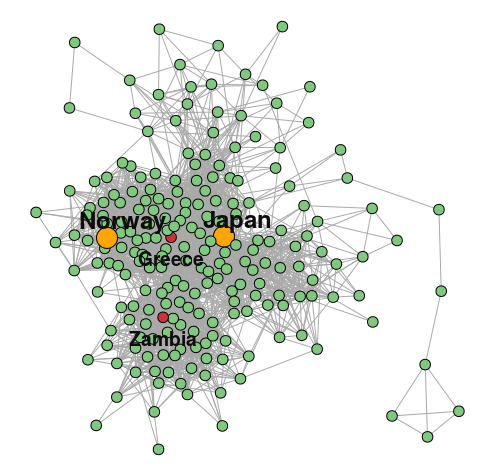I haven’t found a decent measure of the overall connectedness between two nodes, that incorporates all indirect links of all degrees, but that could be an interesting thing to look at.

Another thing we can do with a graph like this is a cluster analysis. A cluster analysis gives us a broader look at the connectedness in the graph by finding groups of nodes that are more connected to each other that to those in the other groups. In other words we are trying to find groups of countries that play against each other a lot.

A simple clustering of the graph gives the following clusters, with some of the country names shown. The clustering algorithm identified 5 clusters that rather perfectly corresponds to the continents. This is perhaps not so surprising since the continental competitions (including the World Cup qualifications) make up a large portion of the data.